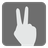Second Index
Elementary+
English RU JA UK

You are given two strings and you have to find an index of the second occurrence of the second string in the first one.

Let's go through the first example where you need to find the second occurrence of "s" in a word "sims". It’s easy to find its first occurrence with a function index or find which will point out that "s" is the first symbol in a word "sims" and therefore the index of the first occurrence is 0. But we have to find the second "s" which is 4th in a row and that means that the index of the second occurrence (and the answer to a question) is 3.

Input: Two strings.

Output: Int or None

Example:

```second_index("sims", "s") == 3
second_index("find the river", "e") == 12
second_index("hi", " ") is None
```